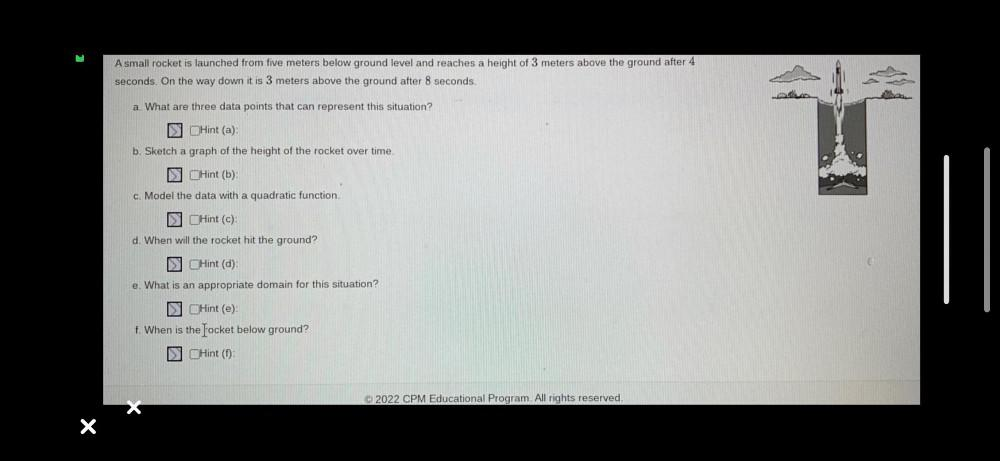Question:

# A small rocket is launched from five meters below ground level and reaches a height of 3 meters above the ground after 4 seconds. On the way down it is 3 meters above the ground after 8 seconds (A) WhA small rocket is launched from five meters below ground level and reaches a height of 3 meters above the ground after 4 seconds. On the way down it is 3 meters above the ground after 8 seconds (A) What are three data points that can represent this situation? (B) Sketch a graph of the height of the rocket over time. (C) Model the data with a quadratic function. (D) When will the rocket hit the ground? (E) What is an appropriate domain for this situation? (F) When is the locket below ground?